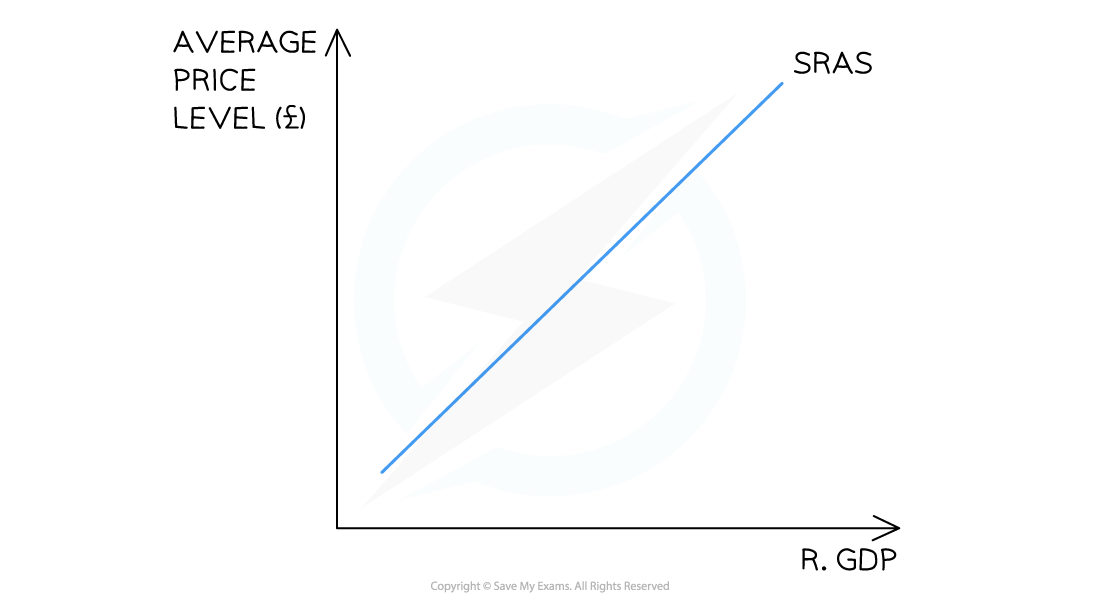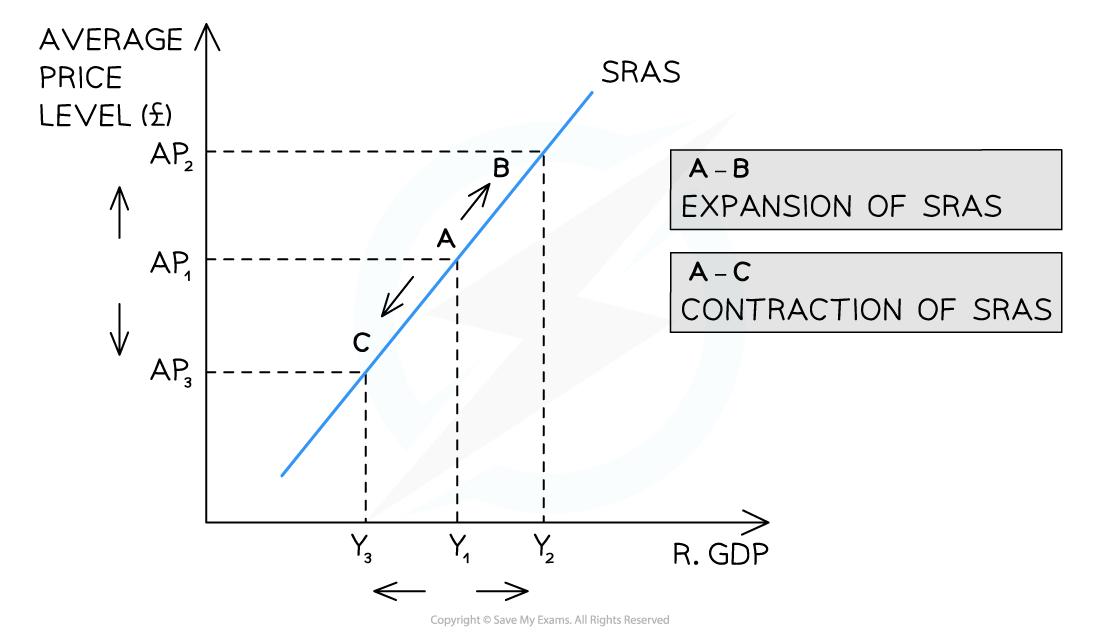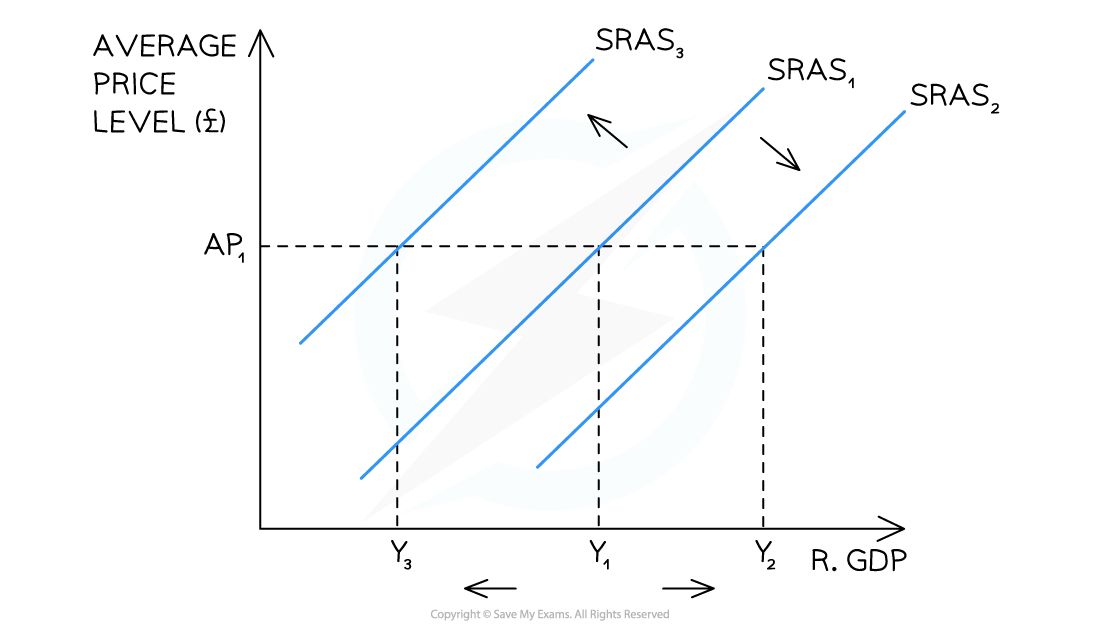# Edexcel A Level Economics A:复习笔记2.3.1 Characteristics of AS

### The Aggregate Supply (AS) Curve

• Aggregate supply is the total supply of goods/services produced within an economy at a specific price level at a given timeA diagram showing the upward sloping short run aggregate supply (SRAS) curve for an economy

• The AS curve is upward sloping due to two reasons
• The aggregate supply is the combined supply of all individual supply curves in an economy which are also upward sloping
• As real output increases, firms have to spend more to increase production e.g. wage bills will increase
• Increased costs result in higher average prices

#### A Movement Along the SRAS Curve

• Whenever there is a change in the average price level (AP) in an economy, there is a movement along the short run aggregate supply (SRAS) curveA diagram showing an increase and decrease in the average price level (AP) which causes a movement along the short run aggregate supply (SRAS) curve leading to a contraction/expansion of SRAS

Diagram Analysis

• An increase in the AP (ceteris paribus) from AP1 → AP2 leads to a movement along the SRAS curve from A → B
• There is an expansion of real GDP from Y1 → Y2
• A decrease in the AP (ceteris paribus) from AP1 → AP3 leads to a movement along the SRAS curve from A → C
• There is a contraction of real GDP (output) from Y1→Y3

#### A Shift of the Entire SRAS Curve

• Whenever there is a change in the conditions of supply in an economy (e.g. costs of production or productivity changes), there is a shift of the entire SRAS curveA diagram showing a shift in the entire short run aggregate supply (SRAS) curve due to a change in one of the conditions of supply in an economy

Diagram Analysis

• A decrease in costs or increase in productivity results in a shift right of the entire curve from SRAS1 → SRAS2
• At every price level, output and real GDP has increased from Y1 → Y2
• An increase in costs or decrease in productivity results in a shift left of the entire curve from SRAS1 → SRAS3
• At every price level, output and real GDP has decreased from Y1 → Y3

### The Relationship Between Short-run & Long-run AS

• Short run aggregate supply (SRAS) is influenced by changes in the costs of production or productivity
• Short run refers to the time period where at least one factor of production is fixed
• Long run aggregate supply (LRAS) is influenced by a change in the productive capacity of the economy
• Productive capacity is changed by changes to the quantity or quality of the factors of production
• When production capacity changes, it is equivalent to a shift inwards/outwards of the production possibilities frontier (PPF)
• Long term economic growth requires the productive capacity to increase